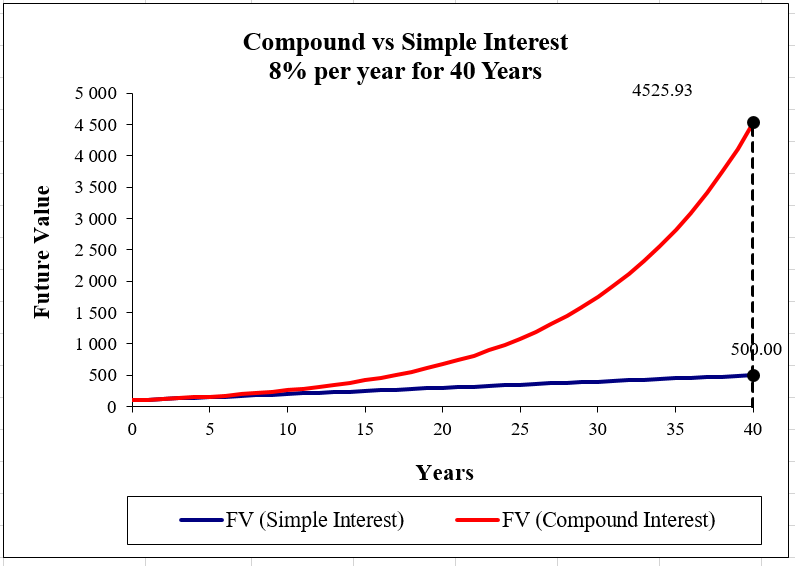# Simple interest vs. compound interest

Want to have financial freedom? One of the best ways to work towards this is to create a savings account and build up a financial safety net. These days, financial institutions make it easier to save money by providing many different options so it's easy to compare savings accounts and find the one that works for you.

When you put money away every month, the interest you earn on it is what really grows it over the long term. Interest is usually split into two categories: simple and compound interest. Let's look at the difference between them.

## What is simple interest?

Simple interest accumulates money only on the original amount you saved and is not as financially beneficial as earning compound interest. Here's an example:

If you put R100 into a savings account for three years and the bank pays 10% interest annually, at the end of the first year the interest you've accumulated will be:
R100 x 10% = R10

Therefore, you'll have R110 in total. Even though you now have more money than you started with, the interest will continue to be calculated only on the original amount. Here's how it works out after three years:

### Year one

R100 x 10% = R10

Therefore, R100 + R10 = R110

### Year two

R100 x 10% = R10

Therefore, R110 + R10 = R120

### Year three

R100 x 10% = R10

Therefore, R120 + R10 = R130

After three years, you'll have R130 in your savings account.

## What is compound interest?

Compound interest is accumulated on the original amount you saved and on the interest already accumulated. Think of it like earning interest on interest. Here's an example:

If you put R100 into a savings account for three years and the bank pays 10% interest annually, at the end of the first year the interest you've accumulated will be:
R100 x 10% = R10

Therefore, you'll have R110 in total, but in the next year, you'll earn 10% on the R110 you've saved, not only on the original R100. Here's how it works out after three years:

### Year one

R100 x 10% = R10

Therefore, R100 + R10 = R110

### Year two

R110 x 10% = R11

Therefore, R110 + R11 = R121

### Year three

R121 x 10% = R12.10

Therefore, R121 + R12.10 = R133.10

## Comparing simple interest and compound interestIn the example above, R100 is saved in two different accounts. The blue line represents simple interest while the red line represents compound interest. Over 40 years, the compound interest has grown by R4 425.93 while the simple interest has grown by R400.

As demonstrated above, compound interest grows your money much faster than simple interest. Simple interest is often used when you take out a loan, such as car finance, which is easier to pay back than a loan with compound interest. Ideally, you'd like to pay simple interest on a loan and accumulate compound interest on savings.

The most important thing to remember is to start saving in the first place. Your money may grow slowly at first, but over the years it will pick up quickly with compound interest. Even a little money saved every month could bring you financial freedom in the long run, so start building up your savings by comparing savings accounts on hippo.co.za today.